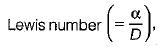Courses

# Test: Fundamentals of Diffusive & Convective Mass Transfer

## 10 Questions MCQ Test Topicwise Question Bank for Mechanical Engineering | Test: Fundamentals of Diffusive & Convective Mass Transfer

Description
This mock test of Test: Fundamentals of Diffusive & Convective Mass Transfer for Mechanical Engineering helps you for every Mechanical Engineering entrance exam. This contains 10 Multiple Choice Questions for Mechanical Engineering Test: Fundamentals of Diffusive & Convective Mass Transfer (mcq) to study with solutions a complete question bank. The solved questions answers in this Test: Fundamentals of Diffusive & Convective Mass Transfer quiz give you a good mix of easy questions and tough questions. Mechanical Engineering students definitely take this Test: Fundamentals of Diffusive & Convective Mass Transfer exercise for a better result in the exam. You can find other Test: Fundamentals of Diffusive & Convective Mass Transfer extra questions, long questions & short questions for Mechanical Engineering on EduRev as well by searching above.
QUESTION: 1

### In a mass transfer process of diffusion of hot smoke in cold air in a power plant, the temperature profile and concentration profile will become identical when

Solution:which relates the temperature field with the concentration field and is ratio of a and D, which govern the transport of energy and mass respectively.

QUESTION: 2

### If heat and mass transfer takes place simultaneously, then the ratio of heat transfer coefficient to mass transfer coefficient is a function of the ratio of

Solution:

Analogy of heat and mass transfer is given by Sh = 0.023 Re0. 8 Sc0.33

QUESTION: 3

### The relative magnitudes of heat and mass diffusion in the thermal and concentration boundary layers are represented by

Solution:
QUESTION: 4

Which of the following relations are true for identical molecular diffusivities of momentum, heat and mass

Solution:
QUESTION: 5

All of the following involves simultaneous heat and mass transfer, except

Solution:
QUESTION: 6

The surface hardening of a mild steel component by the diffusion of carbon molecules is a

Solution:
QUESTION: 7

Schmidt number is ratio of which of the following:

Solution:
QUESTION: 8

Which of the following is a non - dimensional number generally finds application in mass transfer problem

Solution:
QUESTION: 9

Fick’s law of diffusion interms of mass fraction can be written as (where CA = mass fraction)

Solution:
QUESTION: 10

For complete similarity in momentum, heat and mass transfer we should have

Solution: